# Volume 7, 2001, Number 2

Volume 7Number 1 ▷ Number 2 ▷ Number 3Number 4

Some explicit formulae for the composite numbers
Original research paper. Pages 29—31
Full paper (PDF, 256 Kb) | Abstract

Explicit formulae for n-th term of the sequence of all composite numbers and for the sequence of all off composite numbers are proposed.

A note on the values of Zeta
Original research paper. Pages 32—35
Taekyun Kim and Seog-Hoon Rim
Full paper (PDF, 432 Kb) | Abstract

By q-calculation, we construct a q-analogue of Riemann ζ-function, Hurwitz’s zeta function and prove some formulas for the values of these functions. By using these formulae, we can evaluate the values of ζ(5) − Aζ(5, ¼) where A is some rational number.

On Heron triangles, III
Original research paper. Pages 36—47
József Sándor
Full paper (PDF, 1376 Kb)

Expansion of integer powers from Fibonacci’s odd number triangle
Original research paper. Pages 48—59
J. V. Leyendekkers and A. G. Shannon
Full paper (PDF, 1495 Kb) | Abstract

Cubes and squares are expanded in various ways stimulated by Fibonacci’s odd number triangle which is in turn extended to even powers. The class structure of the cubes within the modular ring ℤ4 is developed. This provides constraints for the various functions which help in solving polynomial and Diophantine equations, some simple examples of which are given.

An elementary extension of Hermite’s equality
Original research paper. Page 60
Krassimir T. Atanassov
Full paper (PDF, 96 Kb) | Abstract

Ch. Hermite has introduced the following well known equality for each positive real number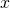and each natural number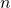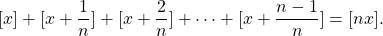We shall extend it, proving that for each positive real numberand each natural numbers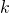and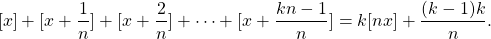On 28-th Smarandache’s problem
Original research paper. Pages 61—64
Mladen V. Vassilev-Missana and Krassimir T. Atanassov
Full paper (PDF, 469 Kb)

Volume 7Number 1 ▷ Number 2 ▷ Number 3Number 4